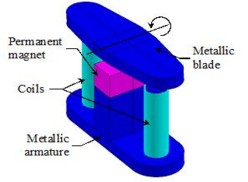# 3D Example : Magneto Static application (tutorial)

## Foreword

This paragraph is a summary of cases treated in detail in the technical document: "Tutorial of magnetostatics".

The files relating to these various cases are available in the documentation directory of the Flux DVD.

## Study carried out

The study proposed in the 3D tutorial "Magnetostatics" is the study of a contactor used to establish or break electric circuits.

## Studied device

The studied device, represented in the figure below, includes the following elements:

• a fixed part composed of a metallic armature, a permanent magnet placed on the top of a central column and two coils placed around two cylindrical lateral columns
• a movable part composed of a metallic blade## Operation

The operation of this device is as follows:

• when either coil is current supplied, the blade moves to the respective coil (rotating motion around an axis in the figure above)
• when the current supply is interrupted, the blade rests immobile as a result of the existence of the magnetic field created by the permanent magnet.

## Studied cases

Three cases are carried out in a Magneto Static application:

• case 1: static study
• case 2: physical parameterized computation
• case 3: geometric parameterized computation

## Case 1

The first case is a static study.

In this study a magnetostatic analysis of the contactor with the movable blade tilted to the left is performed. A geometric parameter α, which allows us to control the angle of the blade around Y-axis, has a fixed value α = -3°. The coils are not current supplied.

## Case 2

The second case is a physical parameterized computation.

In this parameterized study, the physical parameter is the total current supplying one of the coils that varies in the range [– 300 A, + 300 A] with a step of 100 A.

## Case 3

The third case is a geometric parameterized computation.

In this parameterized study, the geometric parameter is the angle α that varies in the range [– 3°, + 3°] with a step of 1°.